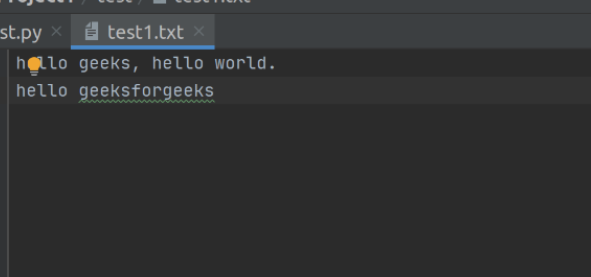GeeksforGeeks App
Open AppBrowser
Continue

# Python program to count the number of blank spaces in a text file

All programs need input to process and after processing gives the output. Python support file handling and allow user to handle files. The concept of file handling has stretched over various other languages, But the implementation is either lengthy or complicated. Python treats file differently as text and binary,
One thing to note while writing data to a file is that its consistency and integrity should be maintained. Once you have stored your data on a file now the most important thing is its retrieval because the computer data store as bits of 1s and 0s and if its retrieval is not done properly then it becomes completely useless and data is said to be corrupted. Hence, writing as well as reading is also an important aspect of File Handling in Python.

### How to count the number of blank spaces or any character?

‘  ‘ (Space) also comes under Printable ASCII Character type,  while NULL is not Printable ASCII Character type.

#### Approach :

How to write to a file using Python?

1. Open a file to write.
2. Count the number of spaces in that text file.
3. Close a file.

Here our text file.Implementation:

Methods #1: Using isspace() function.

Firstly, we will open our text file and store that text file in that variable. A loop is used to count spaces in a text file. if condition ( char.isspace()) to test all the condition, if it returns True then the count will be incremented by 1. After testing all the character’s loop will return False and terminate itself. Finally, the program will display the total number of spaces.

## Python3

 `# this will open the file and store``# in "file" variable``file` `=` `open``(``"test1.txt"``, ``"r"``)`` ` `count ``=` `0``while` `True``:``   ` `    ``# this will read each character``    ``# and store in char``    ``char ``=` `file``.read(``1``)``     ` `    ``if` `char.isspace():``        ``count ``+``=` `1``    ``if` `not` `char:``        ``break`` ` `print``(count)`

Output:

```5
```

Note – isspace() also count new line character therefore it is showing output as 6.

Methods #2: Using Loop:

Firstly, we will open our text file and store that text file in that variable. A loop is used to count spaces in a text file. if condition ( char == ” ” ) to test all the condition, if it returns True then the count will be incremented by 1. After testing all the character’s loop will return False and terminate itself. Finally, the program will display the total number of spaces.

## Python3

 `# this will open the file and store in``# "file" variable``file` `=` `open``(``"test1.txt"``, ``"r"``)`` ` `count ``=` `0``while` `True``:``   ` `    ``# this will read each character``    ``# and store in char``    ``char ``=` `file``.read(``1``)``     ` `    ``if` `char ``=``=` `" "``:``        ``count ``+``=` `1``    ``if` `not` `char:``        ``break`` ` `print``(count)`

Output:

```4
```

Methods #3: Using a python module “functools”.

A Partial function is a function for a particular argument value. They can be created in python by using ‘partial’ from the “functools” library.

## Python3

 `import` `functools`` ` `with ``open``(``"test1.txt"``) as ``file``:``    ``file_char ``=` `functools.partial(``file``.read, ``1``)``     ` `    ``for` `char ``in` `iter``(file_char, ``" "``):``         ` `        ``if` `char ``=``=` `" "``:``            ``count ``+``=` `1`` ` `    ``print``(``"count: "``, count)`

Output:

```4
```

My Personal Notes arrow_drop_up